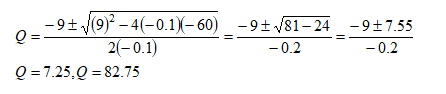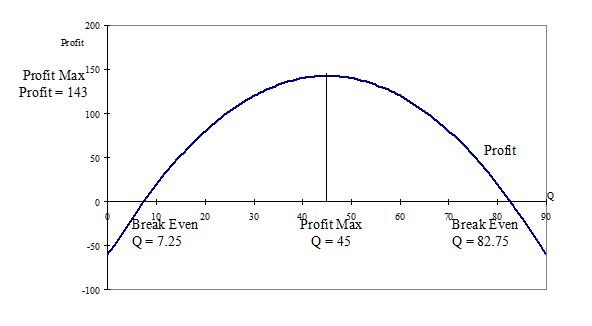In: Economics

# The demand function for a good is given as Q = 130-10P.

The demand function for a good is given as Q = 130-10P.  Fixed costs associated with producing that good are €60 and each unit produced costs an extra €4.

i). Obtain an expression for total revenue and total costs in terms of Q

ii). For what values of Q does the firm break even

iii). Obtain an expression for profit in terms of Q and sketch its graph

iv). Use the graph to confirm your answer to (ii) and to estimate maximum profit and the level of output for which profit is maximised.

## Solutions

##### Expert Solution

i).

TR = P.Q

Q = 130-10P

10P = 130-Q

P = 13-Q/10

TR = (13-Q/10)*Q = 13Q-0.1Q2

TC = FC+VC

TC = 60+4Q

ii).

Firm breaks even where TR = TC

13Q-0.1Q2=60+4Q

-0.1Q2+9Q-60=0

a=-0.1, b=9, c=-60iii).iv).

Profit = TR-TC

Profit = 13Q-0.1Q2-60-4Q=-0.1Q2+9Q-60

Firms break even where total revenue (TR) is equal to total cost (TC).